### IMO Shortlist 2005 problem A1

Kvaliteta:
Avg: 3.0
Težina:
Avg: 6.0
Find all pairs of integers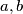$a,b$ for which there exists a polynomial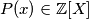$P(x) \in \mathbb{Z}[X]$ such that product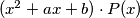$(x^2+ax+b)\cdot P(x)$ is a polynomial of a form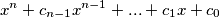where each of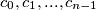$c_0,c_1,...,c_{n-1}$ is equal to$1$ or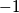$-1$.
Source: Međunarodna matematička olimpijada, shortlist 2005